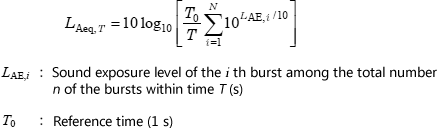Technical Report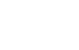What is sound level meter?

10. Methods for measuring equivalent continuous A-weighted sound pressure level (Overview of ISO 1996-1:1982 (JIS Z 8731:1999))

Basic measurement methods for environmental noise are specified in ISO 1996(JIS Z 8731), which, since its establishment on September 18, 1957, has been widely used as the basis for measuring and evaluating noise in Japan.

With the growing awareness of preservation of the global environment in recent years, there has been a trend towards worldwide commonalization of evaluation standards. As part of this, domestic standards have been revised for alignment with ISO standards, including the 1997 revision of the Measurement Act in which phon was replaced with dB, the 1999 enactment of the Environmental Impact Assessment Act, and the 1999 revision of the Basic Environment Act in which L50 was replaced with LAeq.

JIS Z 8731 has also been revised, first in 1983 and, based on ISO 1996-1:1982 and ISO 1996-2:1983, again in 1999. JIS Z 8731 is outlined on the following pages.

10-1 Equivalent continuous A-weighted sound pressure level

Environmental noise consists of multiple sources including trains, automobiles, aircraft and factories. The distribution of these noises is changing every second. ISO 1996(JIS Z 8731) specifies the description and measurement methods for both individual noise sources and the entire group of these noise sources that determine the state of noise at a given location. ISO 1996 (JIS Z 8731) recommends equivalent continuous A-weighted sound pressure level as the basic evaluation measure to control environmental noise and permissible noise limits.

By adopting equivalent continuous A-weighted sound pressure level, ISO 1996(JIS Z 8731)changed dramatically. To determine the permissible level of an existing or planned noise source, Annex 1 stipulates for a given area the collection of acoustic data for appropriate use of the area’s land and the description of noise levels from environmental noise perspectives. Annex 2 describes how to obtain percentile sound pressure level specified in the pre-revision JIS Z 8731:1983 (Methods of measurement and description of A-weighted sound pressure level) for reference purposes, the description and measurement of specific intermittent and impulsive noises, and the method of background noise compensation for steady noise.

10-2 Definitions of terms

The following are the definitions of the terms not covered in Chapter 9, "Sound level meter reading."

(1) Measurement time interval

A period during which the noise is considered to be in a steady state is called an observation time interval. Of an observation time interval, a period during which the noise is actually measured is called a measurement time interval.

(2) Reference time interval

An interval for which an equivalent continuous A-weighted sound pressure level value can be used as the representative value is called a reference time interval, which is decided while taking into consideration the lifestyles of residents in the area being measured and the operating statuses of noise sources. For example, if a day is divided into daytime and nighttime, and the daytime runs from 06:00 till 22:00, the 16 hours between these times are the reference daytime interval. To obtain equivalent continuous A-weighted sound pressure level for that reference daytime interval, ideally the sound pressure level should be measured throughout the 16 hours. In practice, however, a number of intervals are selected within the reference daytime interval for measurement using sampling techniques. For example, if a reference time interval is divided into one-hour segments, each hour is subject to measurement and is called an observation time interval. Sampling techniques can also be applied to observation time intervals. For example, measurements can be taken during part of an observation time interval, like ten minutes from the start of each hour. A period during which equivalent continuous A-weighted sound pressure level is measured continuously is called a measurement time interval. To calculate an equivalent continuous A-weighted sound pressure level of a reference time interval based on data measured that way, the concept of average energy must be used. An extended version of the daily reference time interval is the long-term time interval.

(3) Long-term time interval

If a measured reference time interval noise can be considered as having little long-term fluctuation, and the measurement is to be used as the representative value, the specific period in question is called a long-term time interval.

(4) Long-term average sound level (LAeq, LT)

This is an value which average the series of reference time interval of equivalent noise level in the long-term period over the entire long-term period.

The average value can be calculated as follows.

 (Equation 10-1)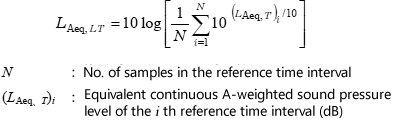(5) Rating level (LAr, T)

When evaluating human reactions to noise, measurements corrected for the specific application can be used as the base quantity. The rating level is an equivalent continuous A-weighted sound pressure level value corrected for the tonality and impulsivity of the subject noise.

 (Equation 10-2)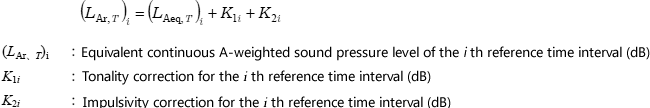Tonality correction K1i may be made if the measured equivalent continuous A-weighted sound pressure level obviously includes auditory-perceived pure tone that is verified by 1/3 octave band analysis and is greater than the adjoining bands by 5 dB or more. Correction of 5 to 6 dB may be made, in which case the correction value must be indicated.
If a specific noise in a time interval is regarded as highly impulsive, the equivalent continuous A-weighted sound pressure level measured in that time interval may be corrected. If the impulsive noise is not continuous but isolated, equivalent continuous A-weighted sound pressure level can be determined while excluding the isolated noise.

(6) Long-term average rating level (LAr, LT)

This is the average rating level over a long-term time interval of the rating levels calculated across a series of time intervals.

 (Equation 10-3)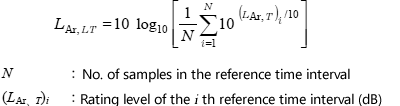10-3 Types of noise

Major changes were made to the classification of noise. While the previous classification was based solely on the fluctuation of noise levels over a period irrespective of noise sources, the latest classification pays close attention to the complex composition of noise sources.

(1) Total noise

Noise measured at a location consists of a range of components from various sources. As such, the level of the compound noise changes in a complex manner over a period. Such compound noise from various sources measured at a location is called total noise, which used to be called environmental noise.

(2) Specific noise

While total noise consists of components from a number of sources, specific noise consists of components selected from among these sources. For example, among various urban noises such as those from transport systems and other daily sources, if we focus on railway noise, that noise is the specific noise in that case.

(3) Background noise and residual noise

If we focus on a specific noise or specific noises among the total noise, noises other than the specific noise(s) of the total noise are collectively called background noise. For example, if we focus on railway noise at a measuring point, road noise from nearby streets is part of the background noise even if it is louder than the railway noise. Residual noise is what is left of the total noise after all specific noises that contribute greatly to the total noise are eliminated from the total noise.

(4) Initial noise

If there has been a change to the local environment, such as the completion of a new road or high-rise building, the total noise before the change is called the initial noise.

(5) Classification of noise based on change over a period of time

Noise can be classified into steady noise, fluctuating noise, intermittent noise and impulsive noise (isolated burst of sound energy and quasi-impulsive noise) based on changes over a period of time. While the previous version of the standard specified a measuring method for each type of noise, the latest standard states that steady noise and fluctuating noise shall be determined by measured equivalent continuous A-weighted sound pressure level and that intermittent noise and impulsive noise shall be determined by equivalent continuous A-weighted sound pressure level calculated based on the duration and sound exposure level of the noise. (Refer to Chapter 3.)

10-4 Sound level meter and calibration

Sound level meters must conform to IEC 61672-1 (JIS C1509-1) for Class 1 precision sound level meters or at least IEC 61672-1 (JIS C1509-1) for Class 2 standard sound level meters. Models capable of measuring equivalent continuous A-weighted sound pressure level, sound exposure level and percentile sound pressure level are convenient.

Sound level meters must be calibrated in the field before and after a set of measuring sessions normally using sound calibrators.

10-5 Point of measurement

When measuring environmental noise, points suitable for measurement must be selected in accordance with any applicable laws that may exist. While points of measurement should be selected or designated based on the purpose, content, site and other conditions of the measurement, generally the following rules must be obeyed.

(1) Outdoors

When measuring ordinary environmental noise, points of measurement must be set up 3.5 m or more away, if possible, from buildings and other objects that reflect sound and 1.2 to1.5 m above the ground to eliminate reflected noise. If it is not possible to set up points of measurement 3.5 m or more away from buildings, etc., it is desirable to measure noise at points as far away as possible from sound-reflecting buildings, etc. If local conditions demand that points of measurement be set up near buildings, etc., this must be stated. The previous standard specified that, when measuring road and other noises on the street, points of measurement must be set up at the end of the sidewalk adjacent to the roadway or, if there is no sidewalk, at the end of the roadway, and also 1.2 to 1.5 m above the ground. On the other hand, the Noise Regulation Act and the Environmental Quality Standards for Noise place importance on points where there are complaints about noise in noise evaluation.

This also applies when evaluating the impact of specific noises such as from factories and entertainment places in the surrounding areas: points of measurement must be selected based on local conditions and in accordance with applicable laws, but foremost at points where there are complaints about noise.

(2) Noise measurement around the building

When evaluating the impact of external noise on a building, points of measurement must be set up 1 to 2 m away from the external walls of the building, etc. and also 1.2 to 1.5 m above the floor level of the story (stories) in question to eliminate any influence of the walls and other sound-reflecting surfaces. JIS Z 8731:1983 specified that, when measuring noise levels in front of a window, a point of measurement must be set up 1 m away from the window on the window’s centerline.

(3) Noise measurement within the building

When measuring noise levels within a building, points of measurement must be set up 1 m or more away from walls and other sound-reflecting surfaces or 1.5 m away from windows and other openings and also 1.2 to 1.5 m above the floor. In most cases, the indoor acoustic field is extremely complex, which makes it desirable to set up multiple points of measurement and to take the average (arithmetic mean or power average) of the measurements.

(4) Noise measurement in work environment

This item was deleted in JIS Z 8731:1999. JIS Z 8731:1983 and the Industrial Safety and Health Act state that, when measuring and evaluating noise of the work environment in factories, offices and other workplaces, points of measurement must be set up at the operator’s ears unless otherwise specified when the operator works mostly at the same place. When the operator does not stay at the same place, points of measurement must be set up at some of the typical points on the route of the operator’s movement and also 1.2 to 1.5 m above the floor. Points of measurement are set up at points of intersection of a 6 meter by 6 meter grid.

(5) Influence of meteorological factors

Noise can be influenced by weather conditions, especially as it travels over a longer distance. If such influence needs to be taken into account, noise must be measured using one of the following methods.

(a) Averaging the results of measurements taken in various weather conditions

Measurement time intervals are set so that a long-term average sound level in various weather conditions at points of measurement can be determined.

(b) Taking measurements in specific weather conditions

Measurement time intervals are set so that the noise being measured is louder, which is normally when wind blows from the source of the noise to the point of measurement.

10-6 Methods for measuring noise

With the Basic Environment Act, the criterion for evaluation was fully changed from median percentile sound pressure level L50 to equivalent continuous A-weighted sound pressure level. With the Noise Regulation Act, the criterion for evaluation remains the percentile sound pressure level L5 for specific facilities but was changed in April 2000 to equivalent continuous A-weighted sound pressure level for automobile noise. The following table shows comparisons between equivalent continuous A-weighted sound pressure level LAeq and percentile sound pressure level L50.

 Table 10-1: Equivalent continuous A-weighted sound pressure level LAeq and percentile sound pressure level L50
Equivalent continuous A-weighted (LAeq) Percentile sound pressure level (L50)
Basic characteristics

· Average energy of noise

· Can be influenced by impulsive and intermittent noise (Low time and spatial stability = High sensitivity)

· Adaptive to fluctuating or compound noise

· Median noise level

· Difficult to be influenced by impulsive and intermittent noise (High time and spatial stability = Low sensitivity)

· Not adaptive to noise with different characteristics or compound noise

· Not suitable for comparison between measurements of different noises

· In noise measurement using both parameters simultaneously, normally LAeq produces higher values than L50 to varying degrees depending on the severity of noise fluctuation.

In relation to residents’ reaction

Reflects exposure levels of intermittent and other noises, resulting in relatively high correlation with residents’ reaction Less reflects intermittent noise exposure levels, resulting in relatively lower correlation with residents’ reaction
Prediction Offers time average of noise energy, making it necessary to only predict the average energy of noise while eliminating the need to reproduce the noise distribution at points of prediction, therefore simplifying and clarifying the prediction calculation Can be influenced by noise distribution, making it necessary for accurate measurement to reproduce the noise distribution at points of prediction, therefore making the prediction calculation difficult (while some predictions were made using empirical formulae)
Measurement Sensitive to noise level fluctuation; when fluctuation is too large, measurement needs to be taken over a longer period of time to obtain stable data (difficult to obtain both reliability and practicality) Stable data can be obtained by measurements of shorter duration
International trend Adopted by many countries and international bodies, facilitating global comparison of data Extremely low worldwide adoption, making global comparison of data difficult

Normally LAeq produces higher values than L50 to varying degrees depending on the severity of noise fluctuation. Generally, the following equations are used to calculate equivalent continuous A-weighted sound pressure level unless otherwise specified.

It is possible to determine equivalent continuous A-weighted sound pressure level by noting data at equal intervals on the level recorder and performing calculations using the data. On the other hand, digital sound level meters are available on the market that are capable of measuring equivalent continuous A-weighted sound pressure level and percentile sound pressure level simultaneously. Using these sound level meters, equivalent continuous A-weighted sound pressure level can be calculated easily.

(1) Fluctuating noise

When measuring noise with severe fluctuation, it is desirable to measure LAeq using integrating sound level meters. In that case, remember to record actual measurement time. Other practical methods include sampling methods and methods using statistical distribution of noise levels.

(a) Sampling method

Equivalent continuous A-weighted sound pressure level can be determined using the following equation based on data sampled between t1 and t2 at Δt intervals.

 (Equation 10-4)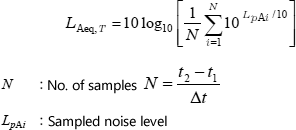When using the equation, note that accuracy may be compromised when the sampling interval (Δ t) is too large relative to the time constant for time weighting. The sampling interval should be 0.25 s or less for Fast time constant and 2 s or less for Slow time constant. When fluctuations are gentle and the measurement time interval is several minutes or more, the sampling interval can be increased up to around 5 s.

(b) Method using statistical distribution of noise levels

Normally, equivalent continuous A-weighted sound pressure level can be determined using the following equation based on statistical distribution of noise level samples divided into 5 dB segments.

 (Equation 10-5)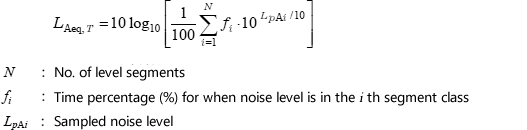If the reading is within 5 dB with A-weighting and Slow time constant used, the noise can be handled as steady noise. The reading can be averaged and the result used instead of determining equivalent continuous A-weighted sound pressure level using integrating sound level meters.

(3) Steady noise with stepped change in level

With steady noise whose level changes in steps and the steps are clearly distinguishable from each other, measure it at each level as steady noise as well as the duration of each level. Then, using the data, equivalent continuous A-weighted sound pressure level can be calculated using the following equation:

 (Equation 10-6)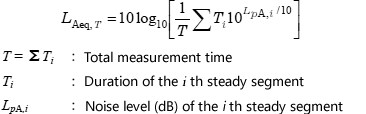(4) Isolated bursts of sound
With bursts of sound that are dominant in the environmental noise, equivalent continuous A-weighted sound pressure level can be determined using the following equation based on the sound exposure level of the bursts within time T.
 (Equation 10-7)